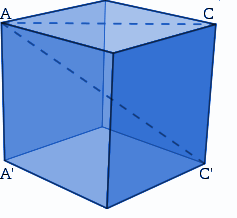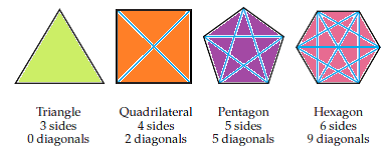# Diagonals

In Mathematics, a diagonal is a line that connects two vertices of a polygon, and whose vertices are not on the same edge. In general, a diagonal is defined as a sloping line or the slant line. The word diagonal is derived from the Greek word “diagonios” which means “from angle to angle”. Also, in matrix algebra, the diagonal of the square matrix defines the set of entities from one corner to the farthest corner. In this article, let us discuss the meaning of the diagonal line, diagonals for different polygons such as square, rectangle, rhombus, parallelogram, etc. with its formulas.

## Diagonal LineA diagonal line is a line segment that connects the two vertices of a shape, which are not already joined by an edge. It does not go straight up, down or across. In other words, a diagonal is a straight line that connects the opposite corners of a polygon through its vertex.

## Diagonal Formula

If “n” is the number of vertices of a polygon, then the number of diagonals of a polygon can be found using the formula:

Number of diagonals of a polygon with “n” vertices = [n(n-3)]/2Let us take an example of a square. A square has 4 vertices. Now, use the above formula to find the number of diagonals of a square.

Number of the diagonals of square = 4(4-3)/2

= 4(1)/2 = 2

Thus, the number of diagonals of the square is 2.

The following table gives the summary of the number of diagonals of different polygons:

 Polygons Number of vertices Calculation Number of Diagonals Triangle 3 [3(3-3)]/2 0 Quadrilateral 4 [4(4-3)]/2 2 Pentagon 5 [5(5-3)]/2 5 Hexagon 6 [6(6-3)]/2 9 Heptagon 7 [7(7-3)]/2 14 Octagon 8 [8(8-3)]/2 20 Nonagon 9 [9(9-3)]/2 27 Decagon 10 [10(10-3)]/2 35

## Diagonal of Square

The diagonals of a square are the line segments that link opposite vertices of the square. A square has two diagonals. The two diagonals of the square are congruent to each other. It bisects each other and divides each diagonal into two equal parts. Each diagonal cuts the square into two congruent isosceles right triangles.

The formula to find the length of the diagonal of a square is:

Diagonal of a Square = a√2

Where “a” is the length of any side of a square.

## Diagonal of Rectangle

A rectangle has two diagonals as it has four sides. Like a square, the diagonals of a rectangle are congruent to each other and bisect each other. If a diagonal bisects a rectangle, two congruent right triangles are obtained.

The formula to find the length of the diagonal of a rectangle is:

Diagonal of a Rectangle = √[l2 + b2]

Where “l” and “b” are the length and breadth of the rectangle, respectively.

## Diagonal of Rhombus

A rhombus has four sides and its two diagonals bisect each other at right angles. If all the angles of a rhombus are 90 degrees, a rhombus is a square or a rectangle. Since all the sides of the rhombus are congruent, and the opposite angles are parallel to each other, the area of the rhombus is given as:

Area of a rhombus, A = (½) pq square units

Where “p” and “q” are the two diagonals of the rhombus.

From the formula of area of a rhombus, we can easily find the diagonal of the rhombus.

Thus, the formula to find the length of the diagonal of the rhombus is:

Diagonal of a Rhombus, p = 2(A)/q and q = 2(A)/p

## Diagonal of Parallelogram

A parallelogram is a quadrilateral. The opposite sides and angles of a parallelogram are congruent, and the diagonals bisect each other. The length of the diagonals of the parallelogram is determined using the formula:

Diagonal of a parallelogram

Diagonal, d1 = p = √[2a2+2b2 – q2]

Diagonal, d2 = q = √[2a2+2b2 – p2]

### Diagonal Solved Examples

Go through the below problems to find the diagonal of a polygon.

Example 1:

Find the diagonal of a square whose side measure is 4cm

Solution:

Given:

Side, a = 4 cm

We know that the formula to find the diagonal of a square is:

Diagonal of a Square = a√2

Now, substitute the side value, we get:

Diagonal, d = 4√2

Now, put the value of √2, which is equal to 1.414

d= 4 × 1.414

d = 5.656.

Thus, the diagonal of a square is 5.656 cm.

Example 2:

Determine the diagonal of a rhombus whose area is 50cm2 and one of its diagonal measures is 7cm.

Solution:

Given:

Area of a rhombus = 50cm2

One of the diagonal of a rhombus, say q = 7cm

Thus, the formula to find the diagonal, p is given as:

Diagonal, p = 2(A)/q

Now, substitute the given values in the formula, we get:

p = 2(50)/7

p = 100/7

p= 14.28, which is approximately equal to 14.3

Hence, the other diagonal of a rhombus is 14.3 cm.

Register with BYJU’S -The Learning App and download the app to learn all the important Maths-related articles and formulas with ease.

## Frequently Asked Questions on Diagonals

### What is diagonal?

A diagonal is a straight line that connects the opposite corners of the polygon through its vertex.

### What is the formula to calculate the diagonal of a polygon?

If “n” is the number of vertices of a polygon, the formula to calculate the number of diagonals of a polygon is [n(n-3)]/2

### What is the length of the diagonal of a square?

The length of the diagonal of the square is a√2, where “a” is the length of any side of a square.

### How many diagonals does a heptagon have?

A heptagon has 14 diagonals, as it has 7 vertices.

### Does a circle have a diagonal?

No, a circle does not have a diagonal, as it has no vertices and sides.